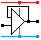Home

rOm
Quest
Glossary

Random
Page
Search
Site
Lush
Sim
Class
Subject
Images

Help
FAQ
Sign
Up
Log
In
 GCSE     Semiconductor     Comparison     Diode     >LED<     MOSFET     Photodiode     Rectifier and PSU     Transistor     Zener Diode

# Semiconductor LED

Site for Eduqas/WJEC - Go to the AQA site.

GCSE    Semiconductors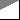Questions 0 to 16   -->  View All

LEDs emit light, when they are forward biased and the applied forward voltage Vf is sufficient for a few milliamps to flow. (About 2 V)

• LEDs are easily destroyed if too much current flows (20mA is a typical maximum).
• LED units used for lighting need a lot more current.
• LEDs are the most efficient affordable light sources.
• LEDs are easily destroyed if the reverse bias voltage ever becomes too big. (As little as 5 Volts might cause damage.)
• LEDs should be protected with a polarity protection diode if signifiant reverse bias voltages are likely.
• Typical red, yellow or green LEDs have about 2 Volts across them but it depends on the colour.
This image shows the photon energies measured in electron-volts.
These values match the forward voltages of LEDs.
•Thanks wikimedia
• Infra-Red: < 1.65 V
• Red: 1.9 V
• Yellow: 2.15 V
• Green: 2.3 V
• Blue: 2.75 V
• Violet: 3 V
• Ultra-violet > 3.27

## LED Circuit

In the circuit below, the resistor limits the current through the LED to a safe level. A typical LED current is 10mA.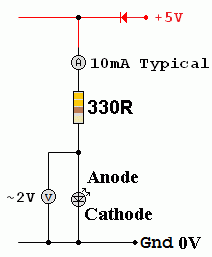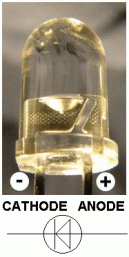## LED Properties

• A typical absolute maximum forward current is 20 mA.
They are more often run at 10mA to give a wide safety margin.
Some new ultra bright LEDs need a lot more current.
• LEDs are much more efficient and reliable than light bulbs.
• LEDs are replacing bulbs in many applications like traffic lights and car brake lights.
• Bicycles use LED lighting for longer battery life.
• Efficient LED torches are for sale too.
• Infrared LED light pulses are used to carry data from remote controls to the TV, DVD player or other device being controlled.
• LEDs are used in fibre optic communications links.
• LEDs are becoming common for domestic lighting because of their high efficiency and low heat generation.
• LEDs are replacing cold-cathode lights for computer and TV flat screen back-lighting.
• Contraflow road works lights now use LEDs. These can be battery powered. The older bulb lights needed a small diesel generator.

## Resistance CalculationThis circuit has a 5 Volt supply. There are 2 Volts across the LED. The remaining 3 Volts are across the resistor. Using Ohm's law ...

• R = V / I
• R = ( 5 - 2 ) / 10mA
• R = 3 / 0.01
• R = 300R

Allowing a small safety margin, the E24 330R was chosen allowing just under 10mA to flow.

## Resistor Power Dissipation CalculationThis circuit has a 5 Volt supply. There are 2 Volts across the LED. The remaining 3 Volts are across the resistor.

• Power = V I

• Power = ( 5 - 2 ) x 10mA

• Power = 3 x 0.01

• Power = 0.03 Watts (30mW)

• This is a very low power so any small resistor would be suitable.

## LED Power Dissipation CalculationThis circuit has a 5 Volt supply. There are 2 Volts across the LED.

• Power = V I

• Power = 2 x 10mA

• Power = 2 x 0.01

• Power = 0.02 Watts (20mW)

## Whole Circuit Power Dissipation CalculationThis circuit has a 5 Volt supply.

• Power = V I

• Power = 5 x 10mA

• Power = 5 x 0.01

• Power = 0.05 Watts (50mW)

## LED Characteristic Curve

The graph below shows the approximate characteristics of an LED.
The reverse leakage current has been exaggerated to make it show on the graph.
The reverse breakdown voltage is quite low (5 volts approx).
It's easy to destroy LEDs. Too much forward current or too much reverse voltage will do the job.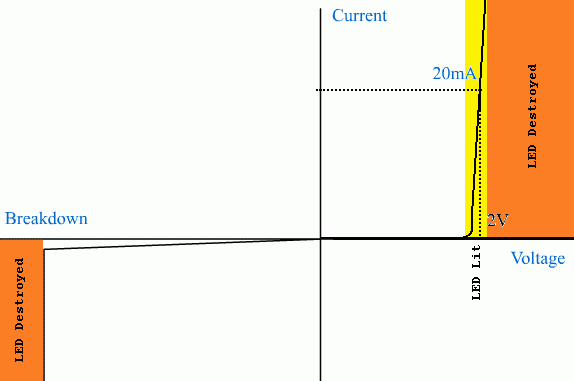## Infra Red LED

This uses Gallium arsenide instead of Silicon as the semiconductor material.## LED Power Indicator - Practical Task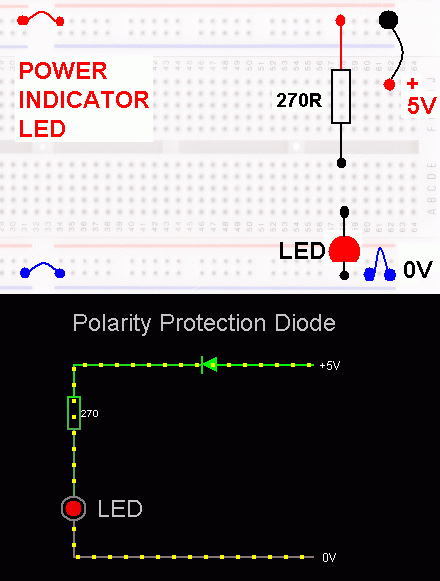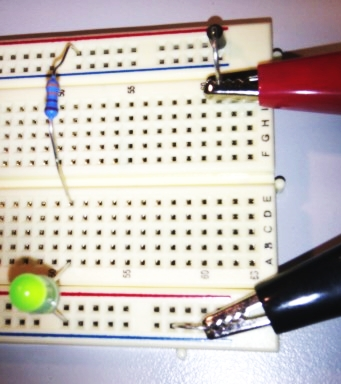Measure ...

1. The voltage across the LED
2. The voltage across the resistor
3. The voltage across the polarity protection diode
4. The power supply voltage
5. The current flowing through the circuit

## LEDs in Parallel - Bad IdeaThis is a bad idea. The current limiting resistor sets the total current which is shared between the LEDs. The same fixed current is split between how ever many LEDs are turned on. One LED might hog the current because the warmest LED will carry the most current so it also stays the warmest. The voltage across LEDs is about two volts. This stays the same if several LEDs are connected in parallel. Connecting a fourth LED in parallel does not increase the total current. It just makes all the LEDs dimmer because the same current is shared between more LEDs.

In this simulation, the LED dimming can be seen but is not obvoius. The current increase is tiny, as expected.

## LEDs in Series - OK

LEDs in series work just fine unless there are too many and the power supply voltage is no longer sufficient.

R = V / I = (12 - 2 - 2 - 2) / 0.01 = 600Ω        Using 560Ω the LED current is 10.7mA. This is safe.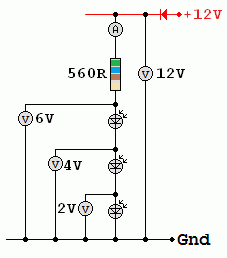Subject Name     Level     Topic Name     Question Heading     First Name Last Name Class ID     User ID

 CloseEscapeX Q: qNum of last_q     Q ID: Question ID         Score: num correct/num attempts         Date Done

Question Text

image url

Help Text
Debug

• You can attempt a question as many times as you like.
• If you are logged in, your first attempt, each day, is logged.
• To improve your scores, come back on future days, log in and re-do the questions that caused you problems.
• In the grade book, you can delete your answers for a topic before re-doing the questions. Avoid deleting unless you intend re-doing the questions very soon.